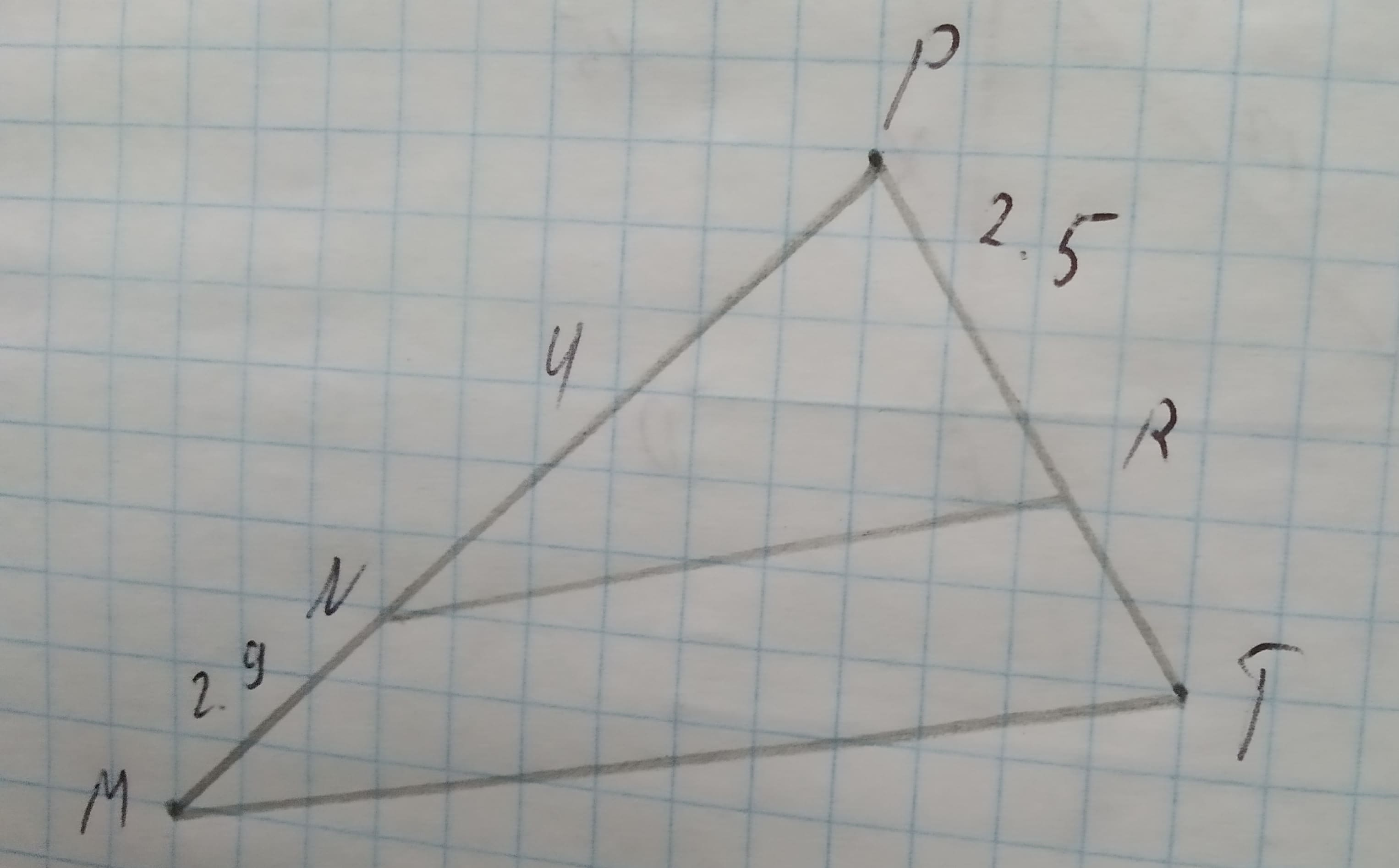# Triangle MPT with bar(NR) || bar(MT) is shown below. The dimensions are in centimeters.12210107121.jpgWhich measurement is closest to the length of bar(RT) in centimeters?1)1.42)1.83)3.44)4.3ankarskogC 2020-11-05 Answered

Triangle MPT with $\stackrel{―}{NR}\mid \mid \stackrel{―}{MT}$ is shown below. The dimensions are in centimeters.Which measurement is closest to the length of $\stackrel{―}{RT}$ in centimeters?
1)1.4
2)1.8
3)3.4
4)4.3

You can still ask an expert for help

• Questions are typically answered in as fast as 30 minutes

Solve your problem for the price of one coffee

• Math expert for every subject
• Pay only if we can solve itRoosevelt Houghton
Step 1
Given,
$\mathrm{△}MPT$ with $\stackrel{―}{NR}\mid \mid \stackrel{―}{MT}$
PN = 4 cm
PR = 2.5 cm
NM = 2.9 cm
We have to find the length of $\stackrel{―}{RT}$ in centimeters.
Step 2
We have
$\stackrel{―}{NR}\mid \mid \stackrel{―}{MT}$
Then, using the properties for two parallel lines cut by transversal lines
$\mathrm{\angle }PNR=\mathrm{\angle }PMT$ ..........(1)
$\mathrm{\angle }PRN=\mathrm{\angle }PTM$ ............(2)
Now,
In $\mathrm{△}NPR\phantom{\rule{1em}{0ex}}\text{and}\phantom{\rule{1em}{0ex}}\mathrm{△}MPT$
$\mathrm{\angle }PNR=\mathrm{\angle }PMT$ (from (1))
$\mathrm{\angle }PRN=\mathrm{\angle }PTM$ (from (2))
$\mathrm{\angle }NPR=\mathrm{\angle }MPT$ (common angle )
So,
From AAA (angle-angle-angle) rule of similarity
$\mathrm{△}NPR\sim \mathrm{△}MPT$
Step 3
Now,
In similar triangle, ratio of corresponding sides will be equal.
$\frac{NP}{MP}=\frac{PR}{PT}=\frac{NR}{MT}$
Taking
$\frac{NP}{MP}=\frac{PR}{PT}$
$⇒\frac{4}{MN+NP}=\frac{2.5}{PR+RT}$ (substituted the values)
$⇒\frac{4}{2.9+4}=\frac{2.5}{2.5+RT}$
$⇒\frac{4}{6.9}=\frac{2.5}{2.5+RT}$
$⇒4\left(2.5+RT\right)=6.9×2.5$
$⇒4RT+10=17.25$
$⇒4RT=17.25-10$
$⇒4RT=7.25$
$⇒RT=\frac{7.25}{4}$
$⇒RT=1.8125$
So,
The lenght of RT = 1.8cm